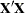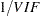Previous Page | Next Page

 The REG Procedure
 Collinearity Diagnostics

When a regressor is nearly a linear combination of other regressors in the model, the affected estimates are unstable and have high standard errors. This problem is called collinearity or multicollinearity. It is a good idea to find out which variables are nearly collinear with which other variables. The approach in PROC REG follows that of Belsley, Kuh, and Welsch (1980). PROC REG provides several methods for detecting collinearity with the COLLIN, COLLINOINT, TOL, and VIF options.

The COLLIN option in the MODEL statement requests that a collinearity analysis be performed. First,is scaled to have 1s on the diagonal. If you specify the COLLINOINT option, the intercept variable is adjusted out first. Then the eigenvalues and eigenvectors are extracted. The analysis in PROC REG is reported with eigenvalues ofrather than singular values of. The eigenvalues ofare the squares of the singular values of.

The condition indices are the square roots of the ratio of the largest eigenvalue to each individual eigenvalue. The largest condition index is the condition number of the scaledmatrix. Belsey, Kuh, and Welsch (1980) suggest that, when this number is around 10, weak dependencies might be starting to affect the regression estimates. When this number is larger than 100, the estimates might have a fair amount of numerical error (although the statistical standard error almost always is much greater than the numerical error).

For each variable, PROC REG produces the proportion of the variance of the estimate accounted for by each principal component. A collinearity problem occurs when a component associated with a high condition index contributes strongly (variance proportion greater than about 0.5) to the variance of two or more variables.

The VIF option in the MODEL statement provides the variance inflation factors (VIF). These factors measure the inflation in the variances of the parameter estimates due to collinearities that exist among the regressor (independent) variables. There are no formal criteria for deciding if a VIF is large enough to affect the predicted values.

The TOL option requests the tolerance values for the parameter estimates. The tolerance is defined as.

For a complete discussion of the preceding methods, refer to Belsley, Kuh, and Welsch (1980). For a more detailed explanation of using the methods with PROC REG, refer to Freund and Littell (1986).

This example uses the COLLIN option on the fitness data found in Example 74.2. The following statements produce Figure 74.49.

```proc reg data=fitness;
model Oxygen=RunTime Age Weight RunPulse MaxPulse RestPulse
/ tol vif collin;
run;
```

Figure 74.49 Regression Using the TOL, VIF, and COLLIN Options
The REG Procedure
Model: MODEL1
Dependent Variable: Oxygen

Analysis of Variance
Source DF Sum of
Squares
Mean
Square
F Value Pr > F
Model 6 722.54361 120.42393 22.43 <.0001
Error 24 128.83794 5.36825
Corrected Total 30 851.38154

 Root MSE R-Square 2.31695 0.8487 47.3758 0.8108 4.89057

Parameter Estimates
Variable DF Parameter
Estimate
Standard
Error
t Value Pr > |t| Tolerance Variance
Inflation
Intercept 1 102.93448 12.40326 8.30 <.0001 . 0
RunTime 1 -2.62865 0.38456 -6.84 <.0001 0.62859 1.59087
Age 1 -0.22697 0.09984 -2.27 0.0322 0.66101 1.51284
Weight 1 -0.07418 0.05459 -1.36 0.1869 0.86555 1.15533
RunPulse 1 -0.36963 0.11985 -3.08 0.0051 0.11852 8.43727
MaxPulse 1 0.30322 0.13650 2.22 0.0360 0.11437 8.74385
RestPulse 1 -0.02153 0.06605 -0.33 0.7473 0.70642 1.41559

Collinearity Diagnostics
Number Eigenvalue Condition
Index
Proportion of Variation
Intercept RunTime Age Weight RunPulse MaxPulse RestPulse
1 6.94991 1.00000 0.00002326 0.00021086 0.00015451 0.00019651 0.00000862 0.00000634 0.00027850
2 0.01868 19.29087 0.00218 0.02522 0.14632 0.01042 0.00000244 0.00000743 0.39064
3 0.01503 21.50072 0.00061541 0.12858 0.15013 0.23571 0.00119 0.00125 0.02809
4 0.00911 27.62115 0.00638 0.60897 0.03186 0.18313 0.00149 0.00123 0.19030
5 0.00607 33.82918 0.00133 0.12501 0.11284 0.44442 0.01506 0.00833 0.36475
6 0.00102 82.63757 0.79966 0.09746 0.49660 0.10330 0.06948 0.00561 0.02026
7 0.00017947 196.78560 0.18981 0.01455 0.06210 0.02283 0.91277 0.98357 0.00568Previous Page | Next Page | Top of Page